# The True Beauty of Extended Kalman Filters

I’ve been working on the Become a Self-Driving Engineer nanodegree for some time now. Recently, as part of the Sensor Fusion module, I was tasked with building an algorithm that can predict a moving car’s trajectory and location given the previous inputs and uncertainty.

# What even is a Kalman filter?

Kalman filters are a special type of algorithm designed to make estimates given uncertain measurements. Hardcoding the algorithms sounds easy and a lot more practical but what makes an estimation algorithm such as a Kalman Filter optimal for such a task is the fact that it takes in indirect and uncertain measurements to make such a prediction.

Sensors such as LiDAR and radar are susceptible to noise and drift. Kalman Filters can merge (fuse) these sensors together to help optimize estimations for positions/detections. That’s exactly what sensor fusion is about. Kalman filters play an integral role in the succession of it.

In the case of autonomous vehicles, Kalman Filters are extensively used in the prediction of motion for other vehicles and pedestrians. Calculating the trajectory and location of an object is crucial to self-driving especially since driving right through can (and will) cause fatalities to other vehicles/pedestrians.

# How Kalman Filters work

Kalman filters follow through a 2 step iteration process: Measurement update and then predictions. It is represented via a series of Gaussian distributions.

Let’s take an example of a 1-D Gaussian below. Let’s say that this is the location of our car:

This is our initial Gaussian estimate of where the car is at time xₖ-₁. Notice that there is a bit of variance σ² but the estimate seems to be pretty confident.

Now let’s predict for time xₖ. Notice at the graph below that our variance σ² is actually even higher than it was before. Let’s now get our new, updated measurement, yₖ:

yₖ is now the measurement update input we get from our sensors. The variance of the measurement update isn’t too much although the root cause of it would mainly be because of the noise from the sensors.

Now, what the Kalman Filter does is that it tries to make an optical state estimate by combing the prediction at xₖ with the updated measurement at yₖ, therefore, producing a Gaussian when an even lower variance than before.

Let’s break down the Kalman Filter algorithm through the 2 main steps — Prediction then Measurement Update:

# Predictions

Given an object's current position and velocity, let’s store that into a vector x. So, in a 1-D world, x = [xₚ,vₚ] where xₚ is the position of the car in the 1-D world with vₚ being the velocity of the car.

We would then have a state transition matrix, F, to incorporate time into the equation. Assuming that vₚ is constant, our state transition matrix would look like:

For our measurement vector, z, we would only incorporate the measurement and not the velocity. This would look like,

This [1, 0] matrix is known as H which is being multiplied by our input position, xₚ, and the velocity, vₚ.

Now that we’ve defined and specified our input matrices, here’s how our position update would look like:

where u is our external motion vector.

The other matrix we’d be using for our predictions is P which is our estimate covariance matrix that helps characterize our uncertainty. This would look like

Remember that F is the state transition matrix and we are taking the transpose of that of which would be multiplied by F and the initial covariance matrix P.

Now that we’ve made our initial predictions, it’s time to make the measurement updates for better predictions of x and P.

# Updating the measurements

We can start making our measurement updates by defining our error vector, y. This is defined as

Remember that z is our measurement input and H is the measurement function (matrix) that eliminates the velocity component of our input vector x.

We would now project our system uncertainty into the measurement space by using our existing measurement function projections by defining that as matrix S.

R is defined as the measurement noise which takes into account the noise of the input sensor.

All of this is actually then mapped into another matrix known as K otherwise known as the Kalman gain.

Now that we’ve defined the Kalman gain, we can simply update our prediction variables, x and P using the following equations:

and updating our estimate covariance matrix P,

This is exactly how we’d program a Kalman Filter for detecting cars and vehicles in the field of autonomous vehicles given all of these inputs.

In an Extended Kalman Filter, we would perform Sensor Fusion by fusing LiDAR and Radar inputs to help make even better predictions than by simply using one than the other.

By combining the strengths of each sensor (LiDAR having high-resolution inputs and Radar providing the velocity input), predictions of an object’s (cars/pedestrians) location and velocity can be made even better, therefore, allowing for accurate estimation and limited fatalities/intervention in real-world situations.

# The real fun begins — Extended Kalman Filters

The Extended Kalman Filter (EKF) uses the main equations/logic that we described above for a basic Kalman Filter. The reason why we’d use an EKF is mainly because of the fact that the predicted state x is mapped to a non-linear function (from the Radar inputs). The goal of an EKF is to solve this issue while being able to fuse the inputs together.

The overall process of the Fusion flow would look like this:

The rundown is that if we’re taking in our initial measurement for any sensor, we’d start by initializing all of our state and covariance matrices. From there, we’d have to then set up the matrices and perform the new predictions + updates. We’d repeat this process until the desired outcome is achieved (ideally avoiding a pedestrian and other objects in the real world). We’d repeat this process for both Radar and LiDAR.

LiDAR is actually very easy to use and program (in the sense of Kalman Filters) because it directly gives us the coordinates required that we can input into the x vector.

Radar is completely different than LiDAR. Let’s break that down a bit more.

Radar uses a completely different coordinate system than the traditional Cartesian system. Radar uses the Polar coordinate system which has a different measurement function. Here’s how z would look:

p is known as the radial distance from the object to the pedestrian. This is essentially the magnitude of the object given that we plot the object’s location and take the vector of that.

φ (rho) is the measured angle between the ray (vector line) and the x-axis. Take a look at the diagram below for more information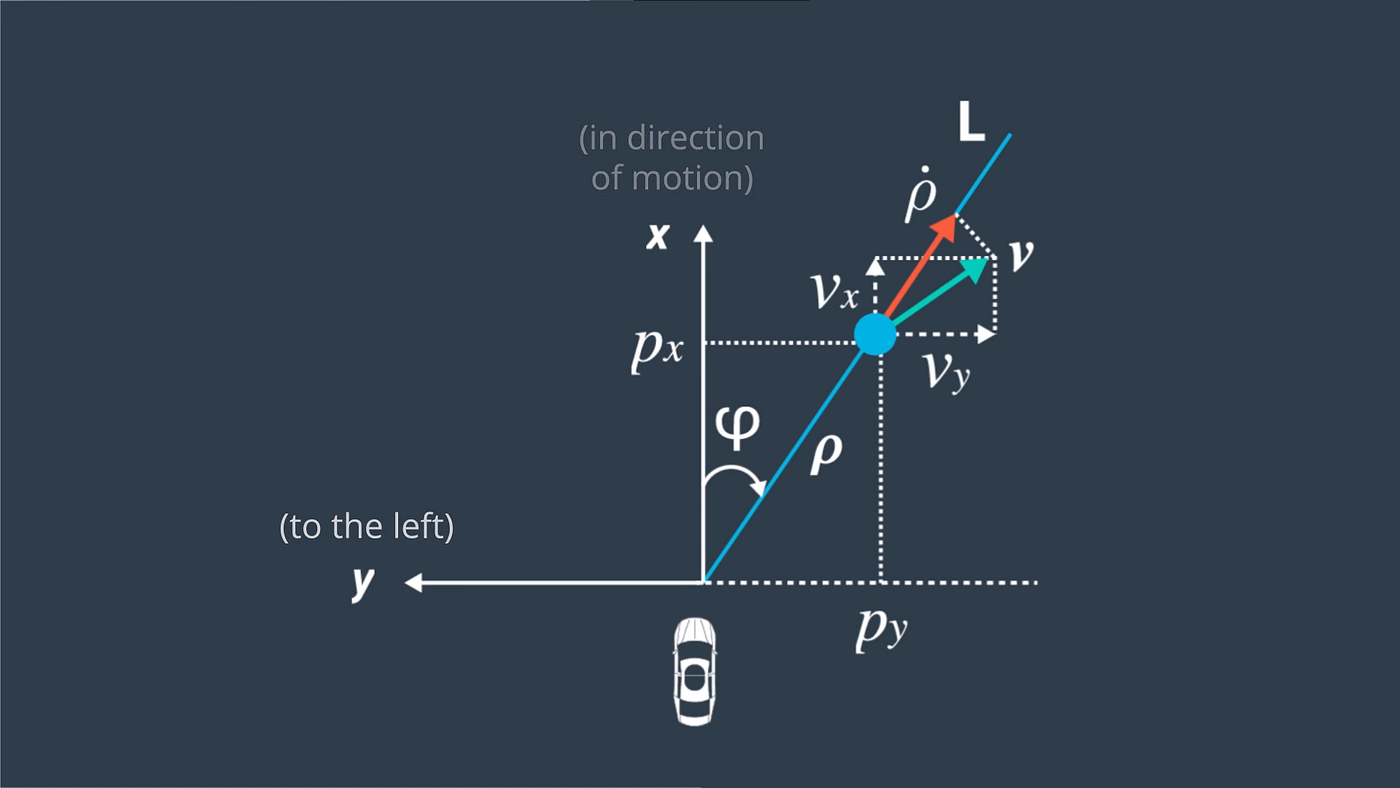Source: Udacity. Note that we always put the object’s location on the x-axis and not the y.

Our Radar noise model would look like something like this:

Notice that we’re taking the variance of every single “coordinate point” for the inputted Radar measurement.

The question that pops up now is: “How do I map from the Cartesian space to the Polar system where we can work with Radar and get the exact measurements of the angles, velocity, and magnitude?” We can do this by defining a function, h(x) to help solve this:

The problem that we get by using such a function to map from Coordinate to Radar space (remember: that’s our measurement vector z) is the fact that h(x) is a non-linear function.

This causes an output that is not Gaussian as a result of not using the non-linear function.

Here’s how it looks like after sampling 10,000 random values from a normal distribution with a mean of 0 through the non-linear function, h(x) = atan(x). Take a look at the image below:

Notice that the graph on the right is not Gaussian. The main problem with this is the fact that Kalman Filters are not applicable anymore — the outputs are not Gaussian since we’re using the nonlinear function of atan(x).

How can we fix this? Let’s take the first-order derivatives (first-order Taylor Series expansion) of the non-linear functions. Here’s how the output looks like:

# Taylor series expansions

Let’s talk a bit more about Taylor series expansions and how this works. Here’s how the general equation looks like:

We can simply replace f(x) for our function, h(x), and apply the same logic. Let’s say that we were applying the first-order Taylor expression to the nonlinear function, arctan(x). What would its derivative (linear function) be?

Let’s say that μ = 0 and σ=3.

So, the function that projects x to z (the measurement space) is

The partial derivative of this would be

When we take the Taylor series equation of this, our linear approximation function output would be

And that would equal

This is exactly how we’d perform First-order Taylor Series equations. Remember that the radar measurements are multi-dimensional equations. How could we work with that?

# Multivariate Taylor Series

The general equation for a multi-dimensional Taylor series expansion is known as

where Df(a) is known as the Jacobian matrix and D²f(a) known as the Hessian matrix where the former represents the first order and the ladder part representing the second-order derivatives of the multi-dimensional equations.

The full Taylor series expression would actually include higher-order terms as well (third, fourth, fifth derivatives, …).

For deriving the linear approximation of h(x), we can simply use the Jacobian matrix ( Df(a) ) since we will assume that (x — a) is a small value.

Let’s talk a bit more about the Jacobian matrix:

# The Jacobian Matrix

The GIST of the Jacobian matrix is this: take all the partial derivatives of 2 variables and store them in a matrix, Hj.

Here’s how Hj would look like for our case:

Here’s how the Jacobian matrix would look like in our case — we would take the derivatives of p, φ, and ṗ with respect to pₓ, pᵧ, vₓ, and vᵧ since our x vector consists of these 4 measurements and we want to convert them into our measurement space, z (which would be the radar coordinates). That’s exactly why the matrix is 3x4–the 3 rows would be part of the measurement space z with the 4 columns being the x vector.

Here’s exactly how Hj would look like after calculating the Jacobian (partial derivatives) of Hj:

# Calculating our final equations for the Extended Kalman Filter

Now that we’ve defined all the matrices and set up everything for making predictions and updating our measurements, we can now put the equations together.

# Prediction equations

Remember that f(x,u) is the function for the Taylor series expansions where x is our input of pₓ, pᵧ, vₓ, and vᵧ and u is the noise in our sensor model.

P’ would be our estimate covariance matrix for uncertainty.

# Measurement update

Remember that we’d have to use the Jacobian matrix Hj instead of the normal H matrix when calculating the system uncertainty projection and the Kalman gain.

Also, note that R is the input measurement noise. We’re also using the h function we defined above to help calculate the error.

# Evaluating the EKF model — RMSE

Now that we’ve defined an Extended Kalman Filter, an effective comparison metric to see how our EKF model performed would need to be in place. We can use the Root Mean Squared Error (RMSE) to help do this exact task.

RMSE can help measure the deviation of the estimated state from the true state.

The difference between the ground truth state (denoted as yᵢ) and the predicted state gives an output known as the residual. It is then squared, averaged, and then square rooted to give the error metric. Note: the lower the error, the higher the accuracy.

I got this all up and running in Udacity’s simulator using C++. Take a look at the Github repository and video provided below to see the code in action.

Udacity simulator result:

# Connect with me

Email

Website

Thanks to Udacity

--

--

--

## More from Analytics Vidhya

Analytics Vidhya is a community of Analytics and Data Science professionals. We are building the next-gen data science ecosystem https://www.analyticsvidhya.com

## Resampling Methods## AutoCorrect / Spell Check using Deep Learning in Python!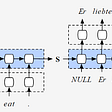## RNN’s and their use## Supercharge Deep Learning (AI) Inference with Amazon Elastic Inference & Amazon SageMaker Neo…## Artificial Neural Networks, Part 5 — Implementing CNN using Tensorflow and Keras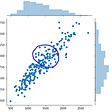## Paper: Wizard of Wikipedia: Knowledge-Powered Conversational Agents## Gender and Race recognition with Transfer, Multi-task Learning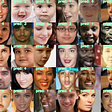## Sri Anumakonda

Building Self-Driving Cars as a 15yo. srianumakonda.com

## Introduction to Self Driving Cars## AUTONOMOUS VEHICLE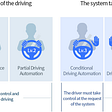## Standing on the Edge, Looking into the Future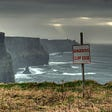## How to avoid car fires